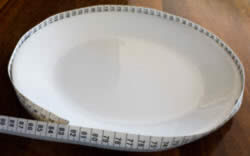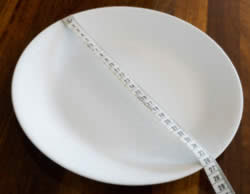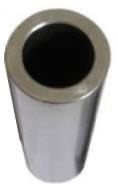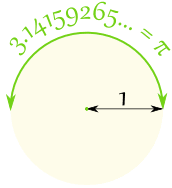# Pi (π)Draw a circle with a diameter (all the way across the circle) of 1

Then the circumference (all the way around the circle) is 3.14159265... a number known as Pi

Pi (pronounced like "pie") is often written using the greek symbol πThe definition of π is:

The Circumference
divided by the Diameter
of a Circle.

The circumference divided by the diameter of a circle is always π, no matter how large or small the circle is!To help you remember what π is ... just draw this diagram.

## Finding Pi Yourself

Draw a circle, or use something circular like a plate.

Measure around the edge (the circumference):I got 82 cm

Measure across the circle (the diameter):I got 26 cm

Divide:

82 cm / 26 cm = 3.1538...

That is pretty close to π. Maybe if I measured more accurately?

## Using Pi

We can use π to find a Circumference when we know the Diameter

Circumference = π × Diameter

### Example: You walk around a circle which has a diameter of 100 m, how far have you walked?Distance walked = Circumference
= π × 100 m
= 314.159... m
= 314 m (to the nearest m)

Also we can use π to find a Diameter when we know the Circumference

Diameter = Circumference / π### Example: Sam measured 94 mm around the outside of a pipe ... what is its Diameter?

Diameter = Circumference / π
= 94 mm / π
= 29.92... mm
= 30 mm (to the nearest mm)

The radius is half of the diameter, so we can also say:For a circle with a radius of 1

The distance half way around the circle is π = 3.14159265...

## Digits

π is approximately equal to:

3.14159265358979323846…

The digits go on and on with no pattern.

π has been calculated to over 100 trillion decimal places and still there is no pattern to the digits, see Pi Normal.

## Approximation

A quick and easy approximation for π is 22/7

22/7 = 3.1428571...

But as you can see, 22/7 is not exactly right. In fact π is not equal to the ratio of any two numbers, which makes it an irrational number.

A really good approximation, better than 1 part in 10 million, is:

355/113 = 3.1415929...
(think "113355", slash the middle "113/355", then flip "355/113")

Summary:

 22/7 = 3.1428571... 355/113 = 3.1415929... π = 3.14159265...

## Remembering The Digits

I usually just remember "3.14159", but you can also count the letters of:

"May I have a large container of butter today"
3 1 4 1 5 9 2 6 5

## To 100 Decimal Places

Here is π with the first 100 decimal places:

 3.14159265358979323846264338327950288 4197169399375105820974944592307816 4062862089986280348253421170679...

### Calculating Pi Yourself

There are many special methods used to calculate π and here is one you can try yourself: it is called the Nilakantha series (after an Indian mathematician who lived in the years 1444–1544).

It goes on for ever and has this pattern:

3 + 4 2×3×4 4 4×5×6 + 4 6×7×8 4 8×9×10 + ...

(Notice the + and pattern, and also the pattern of numbers below the lines.)

It gives these results:

Term Result (to 12 decimals)
1 3
2 3.166666666667
3 3.133333333333
4 3.145238095238
... ... etc! ...

Get a calculator (or use a spreadsheet) and see if you can get better results.### Pi Day

Pi Day is celebrated on March 14. March is the 3rd month, so it looks like 3/14

5839,5050,5052,1745,2106,5473,5477,1744,3236,3237